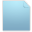###Bitmap 2.0.0 to 3.1.1 upgrade

01 Jul 2016 09:24 #1

Hi~

I used the following codes at chai3d-2.0.0

====================
//global variable
cBitmap* video_grab;
unsigned char* cBuffer;

void RGBCBKAPI FrameCapturedFn_Left (
HWND hWnd,
HRGB hRGB,
void *pBitmapBits,
unsigned long userData )
{

if ( ( pBitmapInfo ) && ( pBitmapBits ) )
{
memcpy((unsigned char*)cBuffer,(unsigned char*)pBitmapBits,cvWidth_left*cvHeight_left*4);
gFrameCount

++;
};

}

// main function

int main(int argc, char* argv[])
{

.......
cBuffer = (unsigned char*)malloc(cvWidth_left*cvHeight_left*4);

video_grab = new cBitmap();
video_grab->m_image.allocate(cvWidth_left,cvHeight_left);
video_grab->m_image.clear(cColorb(0,0,0,255));
video_grab->setPos(0,0, 0);
video_grab->setZoomHV(1, 1);
video_grab->enableTransparency(true);

glutDisplayFunc(updateGraphics);

...........
}

void updateGraphics(void)
{
unsigned char* tBuf;

tBuf = (unsigned char*)(video_grab
->m_image.getData());
//getting pointer to the acutal image data for the 2D bitmap to display
for (y=0;y<cvHeight_left;y++)
for (x=0;x<ex_left;x+=4)
{
tBuf[x+(cvHeight_left-1-(y))*(ex_left)] = cBuffer[x + y*ex_left + 2];
tBuf[x+(cvHeight_left-1-(y))*(ex_left)+1] = cBuffer[x + y*ex_left + 1];
tBuf[x+(cvHeight_left-1-(y))*(ex_left)+2] = cBuffer[x + y*ex_left + 0];
tBuf[x+(cvHeight_left-1-(y))*(ex_left)+3] = 0xff;
}
...
}

======================

I changed them for using chai3d-3.1.1

=======================
int main(int argc, char* argv[])
{

cBuffer = (unsigned char*)malloc(cvWidth_left*cvHeight_left*4);

video_grab = new cBitmap();
video_grab->setLocalPos(0, 0);

video_grab->setZoom(1, 1); // chai3d-3.1.1

video_grab->setUseTexture(true);
video_grab->setUseVertexColors(false);
video_grab->setUseMaterial(false);
video_grab->updateBitmapMesh();
video_grab->setUseTransparency(true);

video_grab->m_texture = cTexture2d::create();
video_grab->m_texture->m_image->allocate(cvWidth_right, cvHeight_right, GL_RGB);
video_grab->m_texture->m_image->clear(cColorb(0, 0, 0, 255));
video_grab->m_texture->m_image->setTransparency(true);

glutDisplayFunc(updateGraphics);

...........
}

void updateGraphics(void)
{

unsigned char* tBuf;
video_grab->m_texture->m_image->erase();
video_grab->m_texture->~cTexture1d();
video_grab->m_texture = cTexture2d::create();
video_grab->m_texture->m_image->allocate(cvWidth_right, cvHeight_right, GL_RGB);
video_grab->m_texture->m_image->setTransparency(true);

tBuf = (unsigned char*)(video_grab->m_texture->m_image->getData());
//getting pointer to the acutal image data for the 2D bitmap to display
for (y = 0; y<cvHeight_left; y++)
for (x=0;x<ex_left;x+=4)
{
tBuf[x+(cvHeight_left-1-(y))*(ex_left)] = cBuffer[x + y*ex_left + 2];
tBuf[x+(cvHeight_left-1-(y))*(ex_left)+1] = cBuffer[x + y*ex_left + 1];
tBuf[x+(cvHeight_left-1-(y))*(ex_left)+2] = cBuffer[x + y*ex_left + 0];
tBuf[x+(cvHeight_left-1-(y))*(ex_left)+3] = 0xff;
}

video_grab->updateBitmapMesh();

}

============================
I add the following to updateGraphics function,
video_grab->m_texture->m_image->erase();
video_grab->m_texture->~cTexture1d();
video_grab->m_texture = cTexture2d::create();

video_grab->m_texture->m_image->allocate(cvWidth_right, cvHeight_right, GL_RGB);

video_grab->m_texture->m_image->setTransparency(true);

If I don't add them, the video_grab image was not updated, in other words, the video_grab shows the first loaded image.

I check the contents of the cBuffer are changed continuously.

Now, the video_grab create cTexture2d every updateGraphics calling. It makes "out of memory" error.

How can I fix this problem??

Thank you~

01 Jul 2016 18:05 #2

Could you perhaps clarify what your application is doing? This will help us better understand the implementation you have posted.

04 Jul 2016 10:26 #3

I would like to display two videos that are outputs of an external device.

The external device has two outputs for stereo left and stereo right view.

1) I connected the external device's outputs to a video capture module of my computer.

2) I stored RGB data captured by the video capture module at cBuffer_left and cBuffer_right, separately, as the following code.
(The function "FrameCapturedFn" is offered by the module's manufacturer)

unsigned char* cBuffer_left;

void RGBCBKAPI FrameCapturedFn (
HWND hWnd,
HRGB hRGB,
void *pBitmapBits,
unsigned long userData )
{

if ( ( pBitmapInfo ) && ( pBitmapBits ) )
{
memcpy((unsigned char*)cBuffer_left,(unsigned char*)pBitmapBits,cvWidth_left*cvHeight_left*4);
gFrameCount++;
};
}

3) I created cBitmap objects for the captured RGB data and attached to camera->m_backLayer.

cBitmap* video_grab_left;

int main(int argc, char* argv[])
{

.......

cBuffer_left = (unsigned char*)malloc(cvWidth_left*cvHeight_left*4);

video_grab_left = new cBitmap();

........
glutDisplayFunc(updateGraphics_left);

........
glutDisplayFunc(updateGraphics_right); // refer to 18-endoscope example.

........
}

4) I copied stored RGB data, cBuffer_, into the cBitmap object, video_grab_ ,at the updateGraphics_ function.

void updateGraphics_left(void)
{
........
unsigned char* tBuf;

tBuf = (unsigned char*)(video_grab_left->m_texture->m_image->getData());
//getting pointer to the acutal image data for the 2D bitmap to display
for (y=0;y<cvHeight_left;y++)
for (x=0;x<ex_left;x+=4)
{
tBuf[x+(cvHeight_left-1-(y))*(ex_left)] = cBuffer_left[x + y*ex_left + 2];
tBuf[x+(cvHeight_left-1-(y))*(ex_left)+1] = cBuffer_left,[x + y*ex_left + 1];
tBuf[x+(cvHeight_left-1-(y))*(ex_left)+2] = cBuffer_left,[x + y*ex_left + 0];
tBuf[x+(cvHeight_left-1-(y))*(ex_left)+3] = 0xff;
}
........
}

At chai3d 2.0.0, video_grab displayed the external device's output almost in real time.

However, at chai3d 3.1.1, video_grab displayed just the first captured image.
The video_grab was not updated.

I don't know why video_grab was not updated.
Anyway, I tried to solve the problem.

I found one solution.

5) I added some codes into the updateGraphics function.

video_grab_left->m_texture->m_image->erase();
video_grab_left->m_texture->~cTexture1d();
video_grab_left->m_texture = cTexture2d::create();
video_grab_left->m_texture->m_image->allocate(cvWidth_right, cvHeight_right, GL_RGB);
video_grab_left->m_texture->m_image->setTransparency(true);

.. (tBuf copy)...
video_grab_left->updateBitmapMesh();

When I added the codes only into the updateGraphics_left function,

the video_grab_left updated the captured images almost in real time,
but the video_grab_right did not.

When I added the codes into both the updateGraphics_left and updateGraphics_right functions,
both the video_grab_left and the video_grab_right updated the captured images,
but the application was finished due to "out of memory" in 2 minutes after started.

I want to know how to update the captured RGB images to display two videos.